Function Repository Resource:

# DirectoryBlock

Temporarily change the current directory for an evaluation and ensure that it is restored when evaluation is completed

Contributed by: Richard Hennigan (Wolfram Research)
 ResourceFunction["DirectoryBlock"][expr] evaluates expr, returning the result and restores any changes to the current or that may have occurred during evaluation of expr. ResourceFunction["DirectoryBlock"][dir,expr] uses dir as the initial working directory when evaluating expr.

## Details and Options

ResourceFunction["DirectoryBlock"] restores the values of and after evaluating expr; it does not prevent changing directory during evaluation.
ResourceFunction["DirectoryBlock"] avoids the need to explicitly use ResetDirectory if using SetDirectory in expr.
ResourceFunction["DirectoryBlock"][dir,expr,CreateIntermediateDirectoriesTrue] will create the directory dir if it does not already exist.

## Examples

### Basic Examples (2)

Evaluate code and restore the current working directory when complete:

 In:=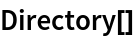Out=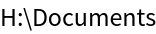In:=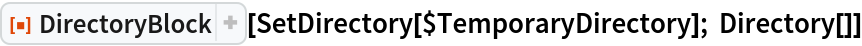Out=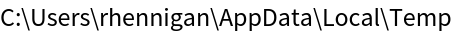Verify that the current directory is restored:

 In:=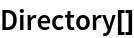Out=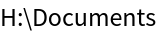Specify a working directory to start from:

 In:=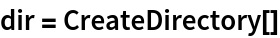Out=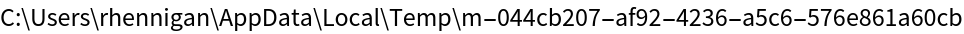In:=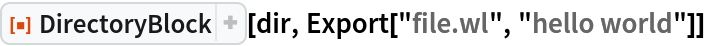Out=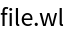The file was created in the specified directory:

 In:=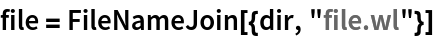Out=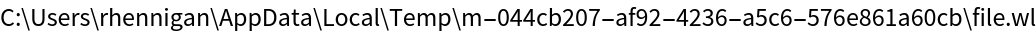In:=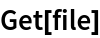Out=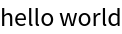### Properties and Relations (4)

SetDirectory pushes a directory onto the :

 In:=Out=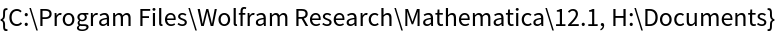Any changes made to during evaluation of DirectoryBlock[expr] are reverted when evaluation of expr completes:

 In:=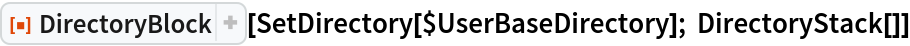Out=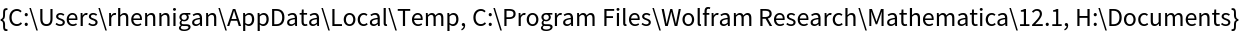Verify that the original directory stack is restored:

 In:=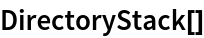Out=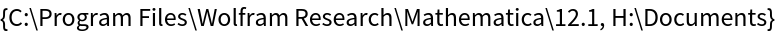Restore the original working directory:

 In:=Out=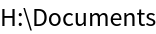Use the resource function ResetDirectoryStack to set the current and back to their initial values:

 In:=Out=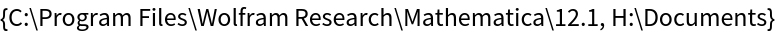In:=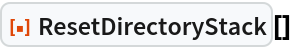Out=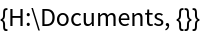Verify:

 In:=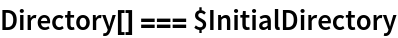Out=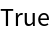In:=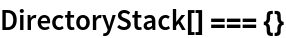Out=DirectoryBlock essentially uses ResetDirectoryStack to restore directory state:

 In:=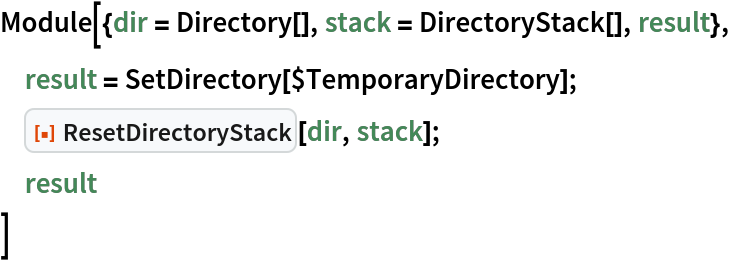Out=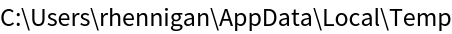In:=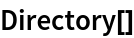Out=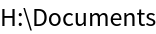In:=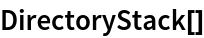Out=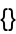Change the current working directory:

 In:=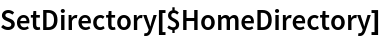Out=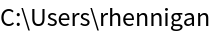Using SetDirectory for both changing to a directory and returning to the original leaves undesirable directories in :

 In:=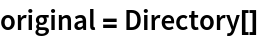Out=In:=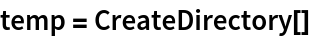Out=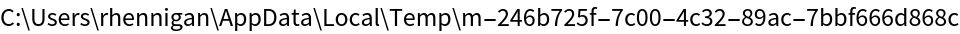In:=This restores the original directory, but leaves extra paths on the :

 In:=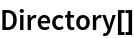Out=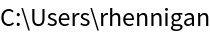In:=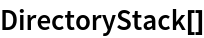Out=In:=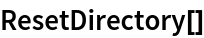Out=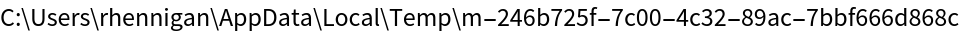In:=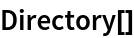Out=Completely reset the :

 In:=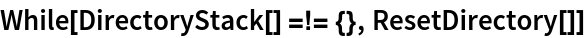Set the current working directory again:

 In:=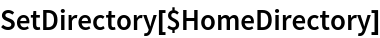Out=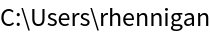Now use DirectoryBlock when changing to the temporary directory:

 In:=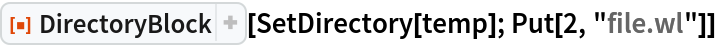Both and are restored:

 In:=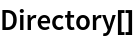Out=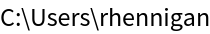In:=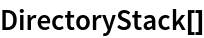Out=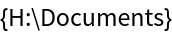This means will work as expected:

 In:=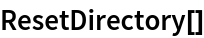Out=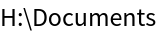In:=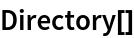Out=## Version History

• 1.0.0 – 24 February 2020